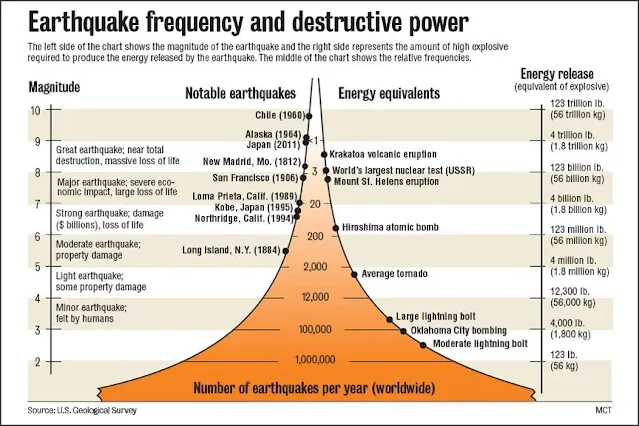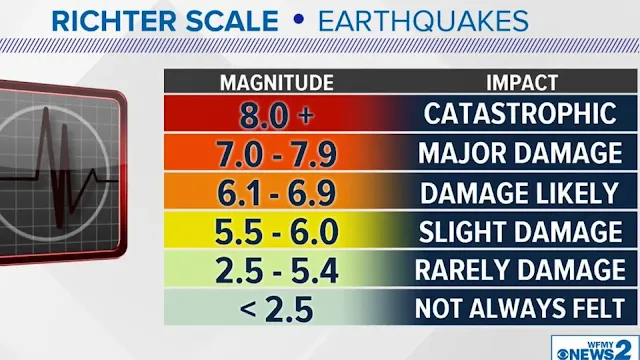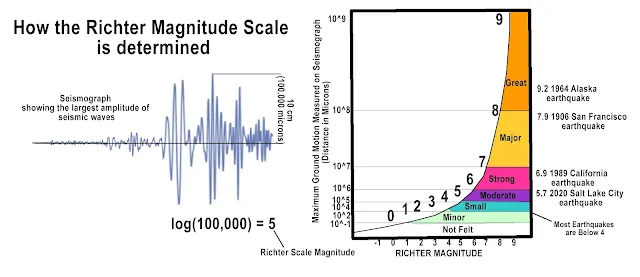# Using the Richter Scale to Measure Earthquakes

## The Richter Scale

The Richter magnitude scale (also Richter scale) assigns a magnitude number to quantify the energy released by an earthquake. The Richter scale is a base-10 logarithmic scale, which defines magnitude as the logarithm of the ratio of the amplitude of the seismic waves to an arbitrary, minor amplitude.

As measured with a seismometer, an earthquake that registers 5.0 on the Richter scale has a shaking amplitude 10 times that of an earthquake that registered 4.0, and thus corresponds to a release of energy 31.6 times that released by the lesser earthquake.

### What Is the Richter Scale?

The Richter scale is a quantitative measure of the magnitude of an earthquake, developed by American seismologists Charles F. Richter and Beno Gutenberg. It is a logarithmic scale, meaning that each increase in magnitude represents a tenfold increase in the amount of seismic energy released.

An earthquake is just what it sounds like - the quaking of Earth. The ground shakes and moves due to rock breaking below the surface. This movement of ground is called seismic activity, and while we can't always tell when, where, or how strongly an earthquake will hit, we can measure its magnitude while it's happening.Using the Richter Scale to Measure Earthquakes

In 1935, Charles Richter developed the local magnitude scale, a scale used to measure the magnitude of earthquakes. Now called the Richter scale, these measurements tell us how much the ground shook during a quake and how much energy was released. This information comes from seismographs, which collect data and directly measure the amount of ground shaking.

### Measuring Ground Shaking

The Richter scale is calculated by measuring the amplitude (height) of the largest seismic wave recorded on a Wood-Anderson torsion seismograph at a distance of 100 kilometers (62 miles) from the epicenter of the earthquake. Adjustments are then made for the distance between the epicenter and the seismograph, as well as for the depth of the earthquake.Using the Richter Scale to Measure Earthquakes

Each increase of one magnitude unit on the Richter scale represents a tenfold increase in the amplitude of the seismic waves, and a 31-fold increase in the amount of energy released. For example, a magnitude 7.0 earthquake is 10 times stronger than a magnitude 6.0 earthquake, and 31 times stronger than a magnitude 5.0 earthquake.

The Richter scale is a base-10 logarithmic scale, which means that each order of magnitude is 10 times more intense than the last one. In other words, a two is 10 times more intense than a one and a three is 100 times greater. In the case of the Richter scale, the increase is in wave amplitude.

The Richter scale runs from 1 - 10 (1 being the least in magnitude and 10 being the greatest), but it is logarithmic. This means that for each 1 point in increase on the scale we get 10 times more ground shaking. Let's look at an example. Say we have a magnitude 1 earthquake on the Richter scale, which is the lowest magnitude earthquake. Compare that with a magnitude 2 earthquake, which is only one step higher (remember, the scale runs from 1 - 10), and you now have 10 times more ground shaking than with the magnitude 1 quake.

Take another step up the scale, so from magnitude 1 to magnitude 3, and this is 10 times more than that first step, so we now have 100 times more ground shaking with just two steps up the scale. That's a lot of seismic activity!

Now let's see how this relates to the total energy released during an earthquake. Through measurements of seismic activity, scientists know that the energy released by an earthquake, which is what causes all that shaking and moving in the first place, increases 32 times for each step up the Richter scale. Take our example from before where we have a magnitude 1 and a magnitude 2 quake. The magnitude 2 quake will have 32 times more energy than the magnitude 1 earthquake.

Now let's compare our magnitude 1 and magnitude 3 quakes. The magnitude 3 quake is two steps up the Richter scale, so we have 32 times more energy for the first step and then 32 times more energy than that up the second step. 32 times 32 gives us about 1,000 times the energy with just two steps up the scale! This is even more amazing when you compare this with the difference in ground shaking. A magnitude 3 earthquake has 100 times more ground shaking than a magnitude 1 quake but about 1,000 times the energy. That's a big difference!How the Richter Magnitude Scale of Earthquakes is determined from a seismograph.

### What do the Numbers on the Richter Scale Mean?

The Richter scale is a logarithmic scale, which means that each increase of one unit on the scale represents a tenfold increase in the amplitude of the seismic wave. For example, an earthquake with a magnitude of 5.0 has a seismic wave that is ten times larger than an earthquake with a magnitude of 4.0.

The Richter scale is an open-ended scale, which means that there is no upper limit to the magnitude of an earthquake. However, the largest earthquake ever recorded had a magnitude of 9.5.

### Richter Scale Magnitude Classification

The Richter scale is divided into the following magnitude categories:

• Micro (less than 2.0): Generally not felt, but can be detected by seismographs.

• Minor (2.0 to 2.9): Often felt, but rarely causes damage.

• Slight (3.0 to 3.9): Noticeable shaking of indoor objects and rattling noises. Minor damage is possible.

• Light (4.0 to 4.9): Moderate shaking and rattling. Minor to moderate damage is possible.

• Moderate (5.0 to 5.9): Strong shaking and ground rolling. Moderate to severe damage is possible.

• Strong (6.0 to 6.9): Severe shaking and ground rupture. Major damage is likely.

• Major (7.0 to 7.9): Very strong shaking and ground rupture. Widespread major damage is likely.

• Great (8.0 or greater): Devastating shaking and ground rupture. Catastrophic damage is likely.

### Limitations of the Richter scale

The Richter scale is a useful tool for measuring the magnitude of moderate earthquakes, but it has some limitations.

• First, the Richter scale is not as accurate for measuring the magnitude of large earthquakes. This is because the Richter scale does not take into account all of the factors that contribute to the magnitude of an earthquake, such as the size of the fault rupture and the duration of the earthquake.

• Second, the Richter scale is only applicable to earthquakes that occur in southern California. Seismologists have developed other magnitude scales for use in other parts of the world.

• Finally, the Richter scale does not measure the intensity of an earthquake. The intensity of an earthquake is a measure of the shaking that is felt at a particular location. The intensity of an earthquake can be affected by a number of factors, such as the distance from the earthquake, the type of ground, and the type of structures in the area.

The Moment Magnitude Scale

The Richter scale was originally designed to measure medium-sized earthquakes, those between magnitude 3 and 7, and within a distance of about 400 miles. The moment magnitude scale was created in 1979 to deal with these issues, but it builds on the Richter scale because it was already so accurate for small- to medium-sized quakes. The moment magnitude scale is the currently accepted scale used to measure medium- to large-sized earthquakes.

The Richter scale remains the standard for quakes smaller than 3.5 in magnitude, and luckily, this is where most earthquakes fall on the scale. About 1,000,000 magnitude 2 earthquakes occur each year, compared with only about 1,000 magnitude 5 quakes. Magnitude 10 earthquakes are extremely rare, occurring less than once a year. Considering that the energy release of a magnitude 2 quake is equal to about 50 kg of explosive while a magnitude 5 releases energy equivalent to 1,800,000 kg, we should be very thankful that they are so infrequent!